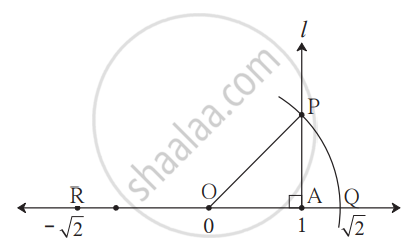# Representation of Irrational Numbers on the Number Line

## Notes

### Representation of Irrational Numbers on the Number Line:

Let us see how we can locate some of the irrational numbers on the number line.

1) Locate √2 on the number line.

Remember that √2 is the length of the diagonal of the square whose side is 1 unit.• On the number line, point A shows the number 1. Draw line l perpendicular to the number line through point A.
Take point P on line l such that OA = AP = 1 unit.
• Draw seg OP. The ΔOAP formed is a right-angled triangle.
By Pythagoras theorem,
OP2 = OA2 + AP2 = 12 + 12 = 1 + 1 = 2.
∴ OP = √2                ....(taking square roots on both sides)
• Now, draw an arc with center O and radius OP. Name the point as Q where the arc intersects the number line. Obviously, distance OQ is sqrt2.
That is, the number shown by point Q is sqrt2.
• If we mark point R on the number line to the left of O, at the same distance as OQ, then it will indicate the number - sqrt(2).
If you would like to contribute notes or other learning material, please submit them using the button below.

### Shaalaa.com

How to represent root 2 and root 3 on number line [00:07:30]
S
0%